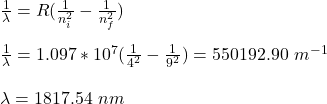Question

For helium, let’s label the emission wavelengths, λ(nf,ni) where nf and ni are, respectively, the principal quantum numbers of the final and the initial states. Calculate the following emission wavelengths: λ(3,4), λ(4,6), λ(4,7) λ(4,8) and λ(4,9).

1.• The emission wavelength for  λ(3,4) is 1875.24 nm
• The emission wavelength for  λ(4,6) is 2625.34 nm
• The emission wavelength for  λ(4,7) is 2165.69 nm
• The emission wavelength for  λ(4,8) is 1944.70 nm
• The emission wavelength for  λ(4,9) is 1817.54 nm

Explanation:

Using Rydberg equation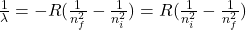where;

R is Rydberg equation = 1.097 x 10⁷ m⁻¹

For λ(3,4)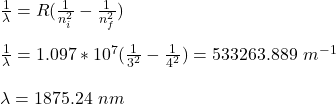For λ(4,6)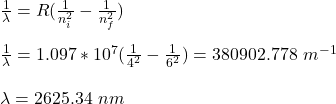For λ(4,7)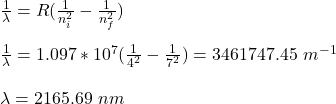For  λ(4,8)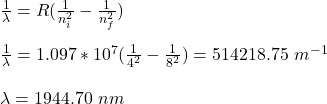For  λ(4,9)